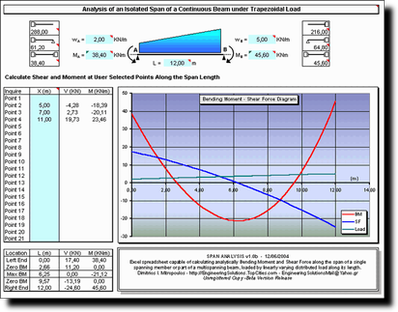.

# Span Analysis

Span Analysis is an Excel spreadsheet capable of analytically calculating bending moment and shear force along a single spanning member or part of a multiple spanning beam, loaded by linearly varying load.
All the information required by the sheet to perform the calculations is the value of the sagging moment and the value of the uniformly distributed load at both ends of the member, and the length of the member. Once all parameters are set, an analytical solution of the bending moment and the shear force diagram is plotted, accompanied with information on the critical points for both graphs. In addition, the values of bending moment and shear force can be exported at the exact point of the user's interest.

It can be used on single spanning structures or members of a continuous beam or a moment resisting frame. The reasons for creating this calculation sheet and its major applications are listed below:

• Identify the analyses action along a single span, once the boundary conditions are calculated using the Moment Redistribution Method (Cross)
• It can be used as a fast calculator sheet for moment and shear along a member for a preliminary design of beams or slabs.This spreadsheet is quite easy to use since all the required data is summarized in five cells. Note that data required cells are colored in sky-blue only.